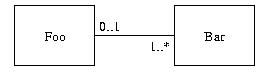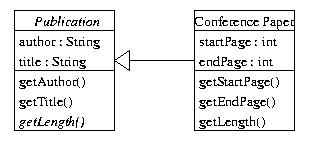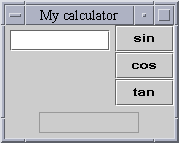CS2000 Software Development
Sample Examination Paper

Answer all questions

Time allowed: Three hours

In answers where you are writing Java programs and you wish to use facilities provided by the standard Java library, you may do so. Do not worry if you cannot remember the exact signatures of library constructors & methods; sensible guesses can score good marks; include comments to explain your intention if you think it might be unclear.

In answers where you are writing Java programs, do not include `javadoc` comments or test-drivers unless the question explicitly asks for them.

1. (48 marks) Do not write your answers onto this examination paper.

1. Which of the following can be used as the basis for a constructor that is to appear in the `Test` class definition:
1. `public void Test() { <statements> }`
2. `public Test() { <statements> }`
3. `public static Test() { <statements> }`
4. `public static void Test() { <statements> }`

Select the most appropriate answer.

2. Which of the following are variables that may be declared in a Java program:
1. class variables
2. local variables
3. instance variables
4. actual parameters
5. formal parameters

Select all correct answers.

3. A programmer is reading about Java strings and arrays, and encounters the following fragment of example code:
```String str = "abc";
System.out.println(str.length());
int[] a = new int;
System.out.println(a.length);
```
The programmer comes to two of the following conclusions. Which ones?
1. For `String`s, `length` is a variable
2. For `String`s, `length` is a method
3. For arrays, `length` is a variable
4. For arrays, `length` is a method

Select both correct answers.

4. Which of the following is a legal return type of a method that overloads the following method:
```public void insert(int a)
{  <statements>
}
```
1. `void`
2. `int`
3. can be anything

Select the most appropriate answer.

5. Which of the following is correct for a method that overrides the following method:
```public void insert(int a)
{  <statements>
}
```
1. the overriding method must return `void`
2. the overriding method must return `int`
3. the overriding method can return whatever it likes

Select the most appropriate answer.

6. What is the effect of attempting to compile and run the following two class definitions that are in separate files:
```public class One
{  public One(String theName)
{  name = theName;
}

public String getName()
{  System.out.println(name);
}

private String name;
}

public class Two
{  public static void main(String[] args)
{  One o = new One("Java");
o.getName();
}
}
```
1. it compiles and runs but produces no output
2. it does not compile
3. it compiles and, when run, displays `Java`
4. it compiles but, when run, gives a run-time error

Select the most appropriate answer.

7. What is the effect of attempting to compile and run the following code:
```public class Test
{  public Test()
{  count++;
id = count;
if (id <= 10)
{  System.out.println(id);
}
}

private int id;
private static int count = 9;

public static void main(String[] args)
{  Test t1 = new Test();
Test t2 = new Test();
}
}
```
1. the compiler reports an error for the assignment `id = count`
2. the compiler reports an error for the declaration of `count`
3. the value 10 is printed twice to the standard output
4. the value 10 is printed once to the standard output

Select all correct answers.

8. What is the effect of attempting to compile and run the following fragment of code:
```int x = 12;
double y = 47.9;
x = y;
```
1. the assignment `x = y` gives rise to a compile-time error
2. the assignment `x = y` gives rise to a run-time error
3. at run-time, `x` will come to contain 47
4. at run-time, `x` will come to contain 48
5. at run-time, `x` will come to contain 47.9

Select the most appropriate answer.

9. What is the effect of attempting to compile and run the following code:
```public class Test
{  public static void main(String[] args)
{  for (int i = 1; i < arrayOfIntegers.length; i++)
{  arrayOfIntegers[i] = i;
}
}

private static int[] arrayOfIntegers;
}
```
1. it compiles and runs, filling the cells of the array with different integers
2. it does not compile
3. it compiles but, when run, throws an array index out of bounds exception
4. it compiles but, when run, throws a null pointer exception

Select the most appropriate answer.

10. A program consists only of the following two class definitions in separate files:
```public class One
{  public int count;
}

public class Two
{  public static void main(String[] args)
{  System.out.println(count);       // a
System.out.println(One.count);   // b
One o = new One();               // c
System.out.println(o.count);     // d
System.out.println(this.count);  // e
}
}
```
Select all lines of the program (a, b, c, d, e) that contain compile-time errors.

11. A polymorphic array of `Object`s is one...
1. ...that can change its length easily
2. ...whose contents can change their type easily
3. ...that can contain values drawn from any of the primitive types
4. ...that can contain references to any Java object
5. ...that can contain references to any object of class `Object` or subclass of `Object`

Select all correct answers.

12. Which of the following can compile?
1. `Object o = new JButton("Press me");`
2. `JButton b = new Object();`
3. `JButton b = new JLabel("Press me");`
4. `JComponent c = new JButton("Press me");`

Select all correct answers.

13. To say that Java uses dynamic method binding means that...
1. ...programmers can easily change the bodies of methods
2. ...programmers can easily change the signature of methods
3. ...the choice of which method to run, for a particular invocation, is made at run-time
4. ...the choice of which actual parameters to use, in a particular method invocation, is made at run-time

Select the most appropriate answer.

14. `One` is an interface, defined as follows:
```public interface One
{  public void insert(int a);

public void delete(int a);
}
```
A class definition, `Two`, begins as follows:
```public class Two implements One
```
Hence, which of the following is true:
1. Class `Two` must include concrete definitions of `insert` and `delete`
2. Class `Two` must include abstract definitions of `insert` and `delete`
3. Class `Two` must include concrete definitions of `insert` or `delete`
4. Class `Two` must include abstract definitions of `insert` or `delete`

Select the most appropriate answer.

15. Using the `One` interface from the previous question, a class definition, `Three`, begins as follows:
```public abstract class Three implements One
```
Hence, which of the following is true:
1. Class `Three` must include definitions of `insert` and `delete` and they must both be concrete
2. Class `Three` must include definitions of `insert` and `delete` and they must both be abstract
3. Class `Three` must include definitions of `insert` and `delete` and they may be either concrete or abstract
4. Class `Three` must include definitions of `insert` or `delete` and they must both be concrete or both abstract.

Select all correct answers.

16. The following program does compile:
```import java.awt.*;
import java.awt.event.*;
import javax.swing.*;
public class Test
{  public static void main(String[] args)
{  JFrame f = new JFrame("A window");
Container contentPane = f.getContentPane();
JButton b = new JButton("Press me");
contentPane.add(b);
b.addActionListener(new ActionListener()
{  public void actionPerformed(ActionEvent ae)
{  count++;
System.out.println(count);
}
});
f.setBounds(100, 100, 100, 100);
f.setVisible(true);
}
private static int count;
}
```
Which of these statements about the execution of this program are true:
1. an event object is created every time the user presses the button
2. any event objects that get created are placed into a queue
3. event objects are passed in as parameters to the handler method of the button's listener
4. event objects are passed in as parameters to the listener method of the button's handler
5. after each press of the button, successively higher integers are displayed on the standard output

Select all correct answers.

17. What is the effect of attempting to compile and run the following code assuming that there is no file called `myfile` on the host file system:
```import java.io.*;
public class Test
{  public static void main(String[] args)
throws IOException
{  DataInputStream dis = new DataInputStream(
new BufferedInputStream(
new FileInputStream(new File("myfile"))));
}
}
```
1. it does not compile because the file does not exist
2. it does not compile because there is no `try/catch` construction
3. it compiles and runs without error
4. it compiles but, when run, it throws an exception that complains about the lack of a `try/catch` construction
5. it compiles but, when run, it throws an exception that complains about the non-existence of the file

Select the most appropriate answer.

18. A file was successfully created and written to using the following fragment of code (plus some exception-handling code that is not shown):
```PrintWriter pw = new PrintWriter(new BufferedWriter(
new FileWriter(new File("myfile"))));
pw.println(356);
pw.close();
```
Subsequently, the file is successfully opened for reading as follows:
```BufferedReader br = new BufferedReader(
new FileReader(new File("myfile")));
```
What is required to read in the contents of the file and store them in a variable of type `int`?
1. four `read` operations, reading 8 bits at a time (followed by 3 shift operations to assemble the bits into a 32-bit word)
2. one `readInt` operation
3. one `readObject` operation, followed by casting to an `int`
4. one `readLine` operation, followed by conversion from a `String` to an `int` using, e.g., `Integer.parseInt`

Select the most appropriate answer.

19. The following is a UML Class Diagram:Which of the following accurately paraphrase parts of the diagram?

1. Objects of class `Foo` need not be associated with corresponding objects of class `Bar`
2. Objects of class `Foo` must be associated with corresponding objects of class `Bar`
3. Objects of class `Foo` must not be associated with more than one object of class `Bar`
4. Objects of class `Foo` may be associated with more than one object of class `Bar`

Select all correct answers.

20. Which of the following are grammatical and true?
1. Java rocks
2. Java sucks
3. Java socks

Select four correct answers.

2. (32 marks)

1. The following UML Class Diagram specifies how one might record an academic's scholarly works:Note that text in italics signifies an abstract class or method.

Give Java definitions for the classes Publication and ConferencePaper.

In both class definitions, include a sensible constructor to initialise all instance variables.

2. Imagine that class definitions for journal papers and books, `JournalPaper` and `Book`, have also been written; both are concrete subclasses of `Publication`.

Write a class method, called `getTotalLength`, that takes in a single parameter and returns an `int`. The parameter is an array that may contain objects constructed from the `ConferencePaper`, `JournalPaper` and `Book` classes (in any mixture), with some possibly empty cells. (You can assume the actual parameter as a whole will not be `null`.) The method will exploit dynamic method binding to compute the total length of the publications in the array.

3. (32 marks)

CD-Direkt is a CD tele-sales company. Customers ring up to place orders for CDs. The order is taken by a sales-person, who records customer details, details of the recipient (if different from the customer) and, the quantity of each CD that the customer wants to order.

Draw a UML Class Diagram based on this description. There is no need to include attributes or operations of classes. Concentrate instead on identifying the classes, their relationships and, in the case of associations, their multiplicities.

Important: The examiners will not answer questions about this description during the examination. If you feel that there are problems with the description (e.g. vagueness, ambiguity or incompleteness), resolve them yourself by stating assumptions in your answer.

4. (48 marks)

Use Swing classes to implement a simple scientific calculator, as follows.

Define a subclass of `JPanel` that contains an editable `JTextField` for entry of a number, three `JButton`s and, below these, an uneditable `JTextField`. When an object is created from your class and added to a visible `JFrame`, something like the following will appear on the screen:When users press one of the buttons, whatever they have typed into the editable `JTextField` is obtained, and converted to a `double` (if possible). The mathematical function that corresponds to the button is then applied to the user's input. The result is displayed in the uneditable `JTextField`.

Include a `main` method for running your program.

Hints & Help.

• The program can have any `architecture' you want. (In particular, the program need not have the Model-View-Controller architecture.)

• Focus on creating components, laying out those components and registering listeners for components that the user can interact with.

You can ignore fine details such as screen size, colours, fonts, etc.

The screenshot is intended to show roughly where you must place the components on the screen, but their precise layout (sizes, co-ordinates, alignment) need not be exactly as depicted.

• The code for converting a `String` stored in variable `str` to a `double` is:
```double number = Double.valueOf(str).doubleValue();
```
A `NumberFormatException` is thrown if the `String` cannot be converted to a `double`.

• Assume that you can use three class methods from the `Math` class to carry out the computations you need, `Math.sin, Math.cos` and `Math.tan`. (Ignore problems that might arise with these methods, such as taking the tangent of 90.)

• Should you wish to display an error message in a separate little window on the screen, this can be done with the following:
```JOptionPane.showMessageDialog(
null,
"Your error message",
"A title for the little window",
JOptionPane.ERROR_MESSAGE);
```

© Derek Bridge, Department of Computer Science, University College Cork. All Rights Reserved.
d.bridge@cs.ucc.ie